# IB DP Physics: HL复习笔记12.1.8 The Wave Function

### The Wave Function

• Bohr showed that the angular momentum of an electron orbiting a hydrogen atom was quantised
• He also showed that the allowed electron energies were also quantised
• Unfortunately, this original model only worked for hydrogen
• Schrodinger then developed an equation that could be used to calculate the quantised energy levels for other atoms
• The only information the equation needs is the shape of the particle’s potential energy function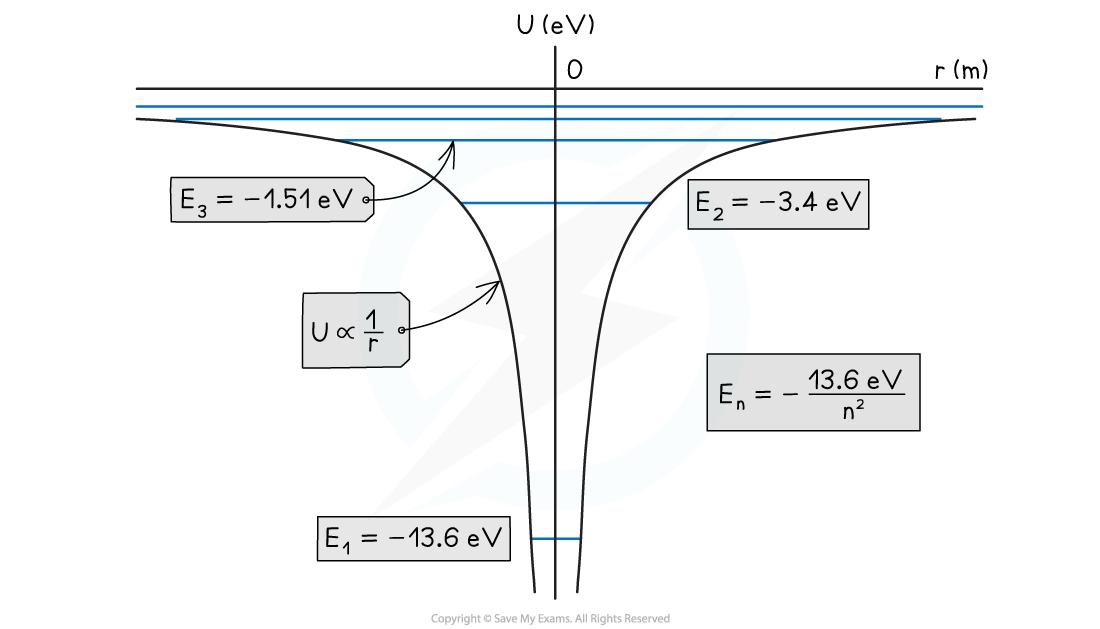The potential energy curve for hydrogen and the quantised energy levels for n = 1, 2, 3, etc

• On the potential energy curve for hydrogen:
• The higher the energy levels, the closer together they become in energy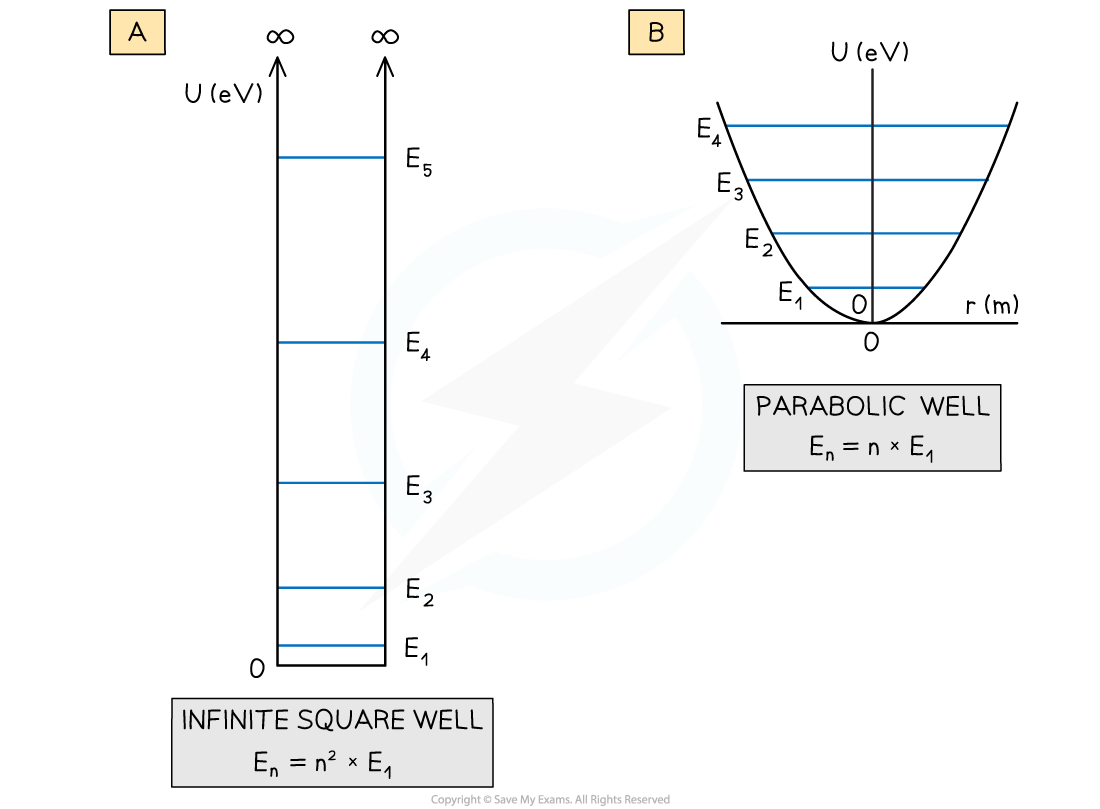The potential energy curves for (a) an infinite square well and (b) a parabolic well and their associated quantised energy levels

• The shapes of these graphs also produce quantised energy levels but with a different energy distribution
• For the parabolic well, the energy levels are equally spaced
• For the infinite square well, the energy levels at larger energies are further apart – this is different to the case for hydrogen
• Finally, as the infinite square well gets wider, the quantised energy levels get closer together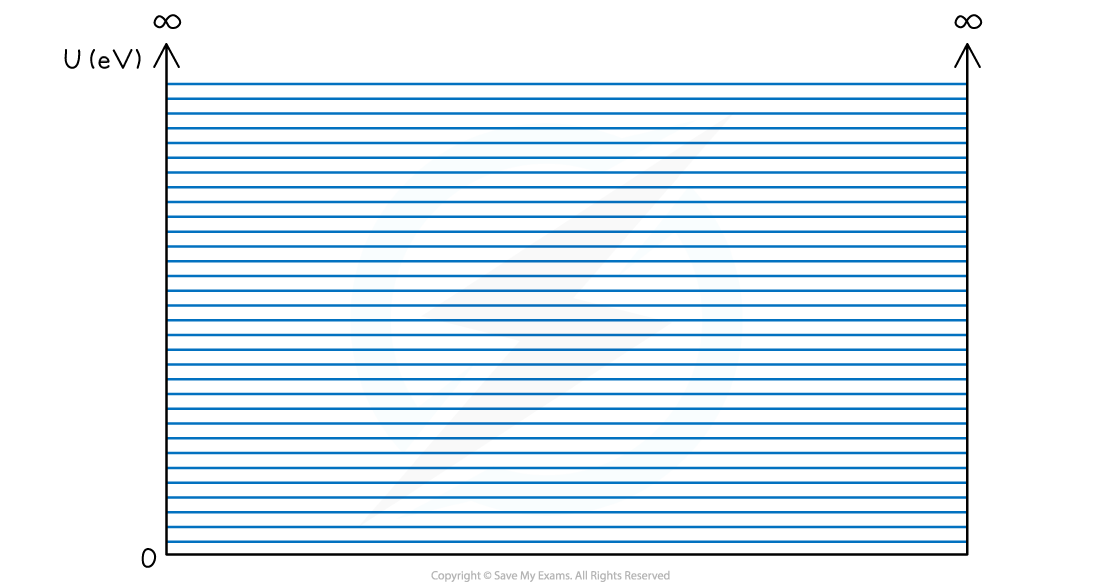The "swimming pool" square well where the width is very large. For a wide well, the quantised levels get so close together that the allowed levels are effectively “continuous”

• The Schrodinger equation predicts not only the distribution of energy levels for a particular potential energy curve
• Each energy level also has a wavefunction, usually given the symbol Ψ (Psi)
• Wavefunctions of the infinite square well are shown below
• They look similar to standing waves on a stretched string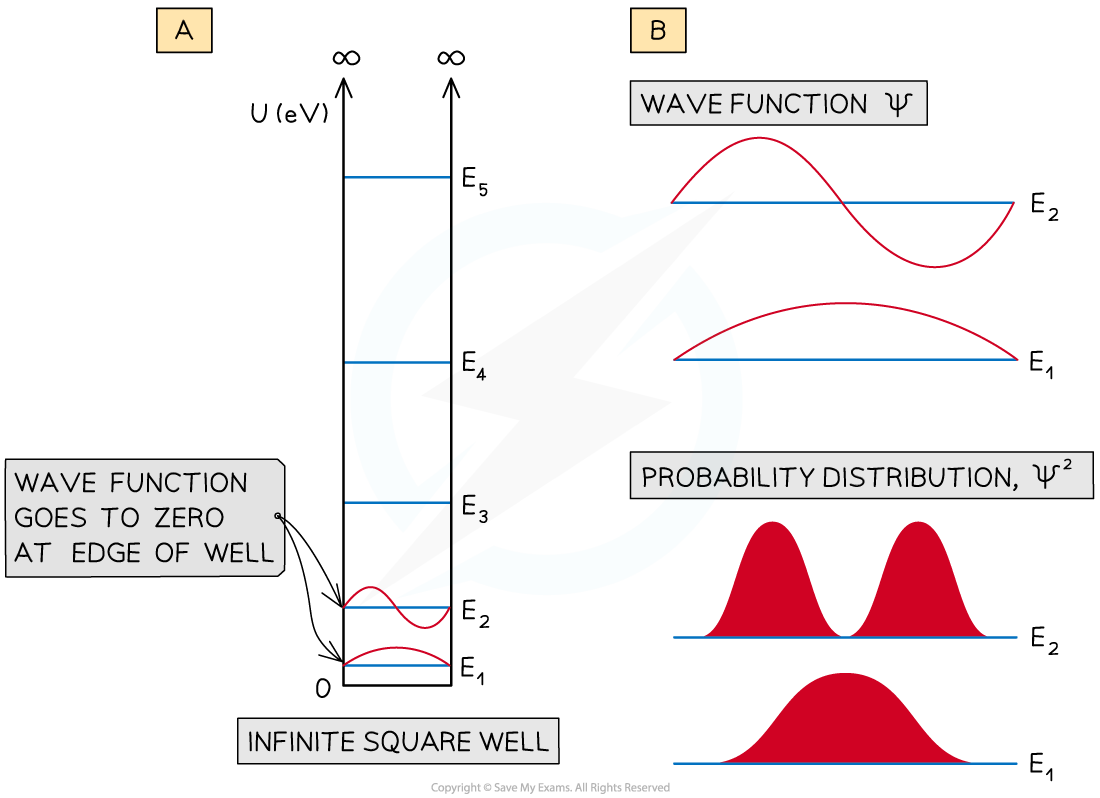(a) Wave functions for E1 and E2 of an infinite square well. (b) an expanded view of the wavefunctions Ψ, and the probability

• The physical significance of the wavefunction Ψ is that:
• The square of the wavefunction, Ψ2 dr gives the probability of finding the particle in the region of width dr
• The wavefunction is said to be normalised
• This means the probability of finding a particle somewhere in the well at a particular energy level is equal to one
• The infinite square well shows the wave function going to zero at the edges of the well
• This means that the probability of finding the particle close to the walls of the well is small
• For the energy level E1 the probability of finding it at the centre of the well in large
• However, for E2 the probability of finding it at the centre of the well is small
• A different result occurs for a square well that is not infinitely deep
• For two energy levels in a square well, the wave functions penetrate the barrier regions as decaying exponential curves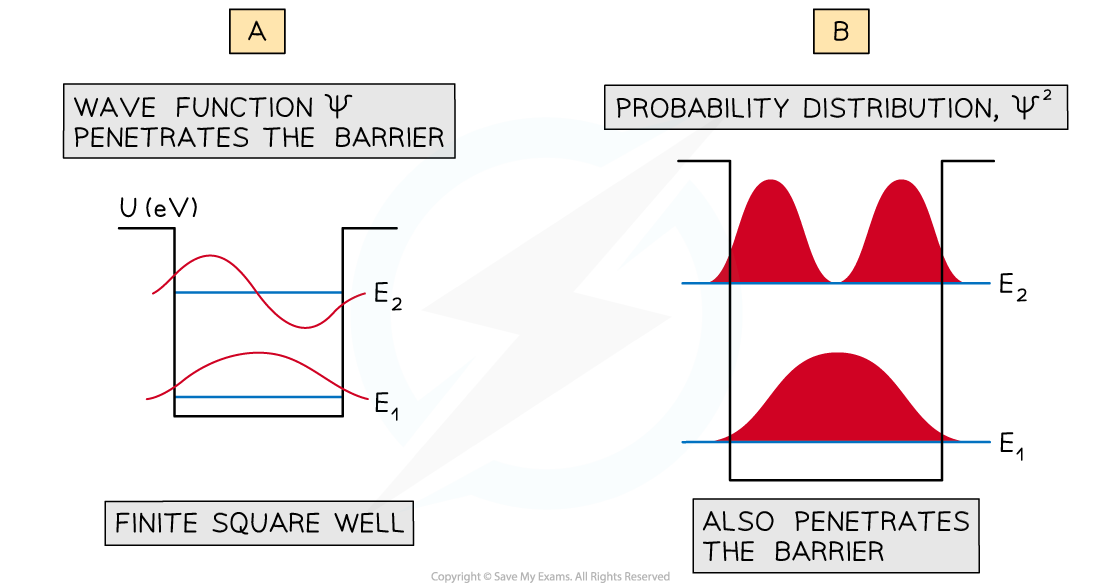(a) Wave functions for E1 and E2 for a finite square well. (b) the wavefunctions Ψ, and the probability distribution Ψ^2 are seen to penetrate the barrier

• This means that the probability of finding the particle in the classically forbidden barrier region is not zero